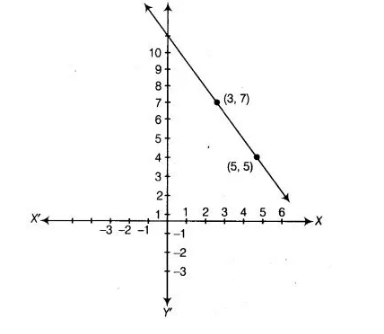# Draw the graph of the linear equation`
Question:

Draw the graph of the linear equation whose solutions are represented by the points having the sum of the coordinates as 10 units.

Solution:

As per question, the sum of the coordinates is 10 units.

Let x and y be two coordinates, then we get x + y = 10.For x = 5, y = 5, therefore, (5, 5) lies on the graph of x + y = 10.

For x = 3, y = 7, therefore, (3, 7) lies on the graph of x + y = 10.

Now, plotting the points (5, 5) and (3, 7) on the graph paper and joining them by a line, we get graph of the linear equation x + y = 10.